# SSC CGL EXAMS 2018 | Reasoning Ability Practice Questions (Day-15)

Dear Aspirants, Here we have given the Important SSC Exam 2018 Practice Test Papers. Candidates those who are preparing for SSC 2018 can practice these questions to get more confidence to Crack SSC 2018 Examination.

1) Raghul’s birthday is on Friday 14th may. On what day of the week will be Monisha’s birthday in the same year if monisha was born on 29th August?

a) Monday

b) Tuesday

c) Wednesday

d) Sunday

2) In certain code, SOFT is coded as PRCW, PINK is coded as MLKN, then how WING is coded as

a) TXJK

b) ZKJL

c) TLKJ

d) LZKJ

3) If ‘+’ means ‘’, ‘×’ means ‘÷’, ‘- means ‘×’, and ‘÷‘’means ‘+’, then find the value of

15 × 5 – 6 + 7 ÷ 4?

a) 10

b) 11

c) 15

d) 20

4) Find the missing number from the given response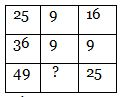a) 9

b) 16

c) 36

d) 12

5) In the question two statements are given, followed by conclusions, I and II. you have to consider the statements to be true even if it seems to be at variance from commonly known facts. You have to decide which of the given conclusions, if any, follows the given statement

Statement:

Some Politics are Constitution

Some constitutions are not illicit

Conclusion:

I. Some illicit is constitution

II. No constitution is illicit

a) Only I Follow

b) Only II follow

c) Either I or II follow

d) Neither I nor II follow

6) Three position of dice are shown below, which of the value is opposite to %  in the given dice?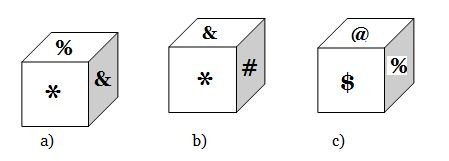a) &

b) #

c) \$

d) @

7) Which answer figure will complete the pattern in the question figure?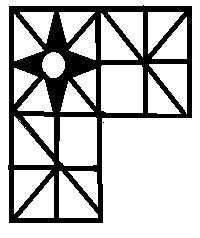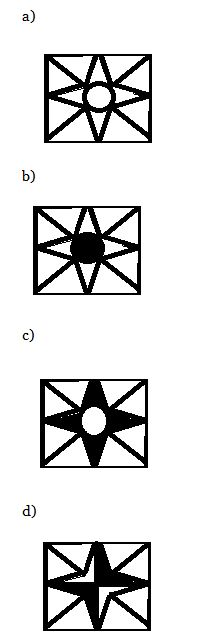8) In following questions, select the related word from given response

Slovakia: Bratislava : : Slovenia : ?

a) Djibouti

b) Ljubljana

c) Reykjavik

d) Nuuk

9) In following questions, select the related number from given response

7 : 350 : : 8 : ?

a) 510

b) 500

c) 520

d) 550

10) Hema pointing to a guy, and said to his friend that“ He is the only son of husband of  my mother’s in law”. Then how Hema is related to that guy?

a) Sister

b) Sister in law

c) Niece

d) Wife

19th May – Friday

Days left in may = 14

Day in June = 30

Days in July = 31

Days in August = 25

Total days = 100/ 7

Remaining 2 days

So, after 2 days will be Sunday. Monisha’s birthday is on Sunday.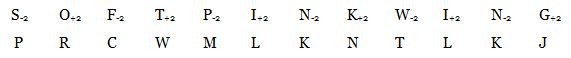15 × 5 – 6 + 7 ÷ 4

On converting as coded

(15 ÷ 5) × 6 – 7 + 4 =

3 × 6 – 7 + 4 = 18 – 7 + 4 = 15

√25 + √ 16 = 5 + 4 = 9

√36 + √ 9 = 6 + 3 = 9

√ 49 + √ 25 = 7 + 5 = 12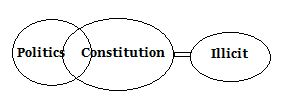By observing the figures a) and b) % is adjacent to @, \$, * and &. Thus, # must be the opposite of %.

73 + 7 = 350

83 + 8 = 520

Husband of Hema’s mother’s in law is Hema’s father in law. Her father’s in law only son is Hema’s Husband. Thus Hema is wife of that guy.

*********************************